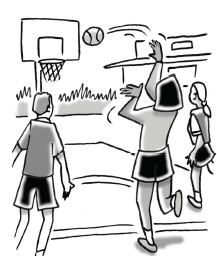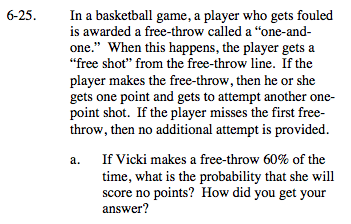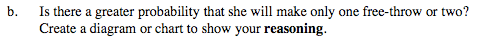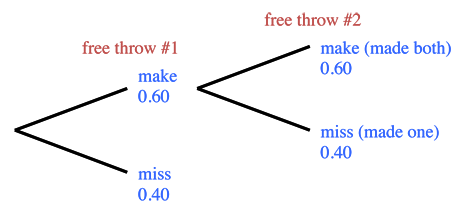Home > GC > Chapter 6 > Lesson 6.1.3 > Problem6-25

6-25.
1.In a basketball game, a player who gets fouled is awarded a free-throw called a “one-and-one.” When this happens, the player gets a “free shot” from the free-throw line. If the player makes the free-throw, then he or she gets one point and gets to attempt another one-point shot. If the player misses the first free-throw, then no additional attempt is provided. Homework Help ✎

1. If Vicki makes a free-throw 60% of the time, what is the probability that she will score no points? How did you get your answer?

2. Is there a greater probability that she will make only one free-throw or two? Create a diagram or chart to show your reasoning .The sum of the probabilities of all the possible outcomes is always 1.Use a tree diagram.Calculate probability:
P(one point) = (0.6)(0.4) = 0.24
P(two points) = (0.6)(0.6) = 0.36